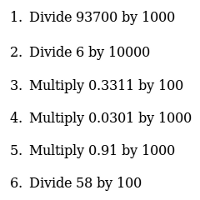# Multiplying And Dividing Powers Of Ten

### Generate as many printable worksheets as you like

By

My son had trouble multiplying and dividing by ten, a hundred, a thousand, and so forth. When I sat down with him, I discovered the cause - he was using an outdated method.

In grade 1 or 2, he would have picked up the rule :

To multiply by 10, you add a zero at the end

Now, that works fine for numbers like 3, 12 or 1739. To multiply these by zero, you really do add a zero on the end - getting 30, 120 and 17390. However, the rule fails you when you need to multiply decimals - add a zero onto the end of 19.47 and you get 19.470, not the right answer at all.

I taught him to imagine, instead, that a number like 19.47 has an invisible infinite string of zeroes before and after - as if it were ...00000019.47000000..., say - and even a number like 3 has the same, as well as an invisible decimal place, making it ...0000000003.00000000...

Then, I taught him a new rule :

To multiply or divide by a power of 10, you shift the decimal point

This rule is just as simple as the earlier one (once you know what a decimal point is), but it works for all kinds of numbers. So, to multiply 19.47 by 1000, he'll now shift the decimal place three spaces across, writing in any invisible zeroes he needs. So :
...00000019.47000000... ~> ...000000194.7000000... ~> ...0000001947.000000... ~> ...00000019470.00000...

Or, to divide by 1000, he'd shift the decimal point three places to the left, so :
...00000019.47000000... ~> ...0000001.947000000... ~> ...000000.1947000000... ~> ...00000.01947000000...

Hopefully, this new rule will set him straight. My wife asked me to prepare some power of 10 multiplying and dividing practice questions, and I thought - I'm not going to be the only parent who wants a worksheet like that. So, instead of making one worksheet for my son, I made a worksheet generator. Now anyone who wants can make and print as many worksheets as they like for multiplying and dividing numbers by powers of ten. Use the form below, click 'Go!', and then print out the results. I hope it helps you and the kids you are trying to help!

 Multiplying And Dividing By Powers Of Ten Printable Worksheet Generator How Many Questions? Maximum Power? 10^ Maximum Number Of Digits?